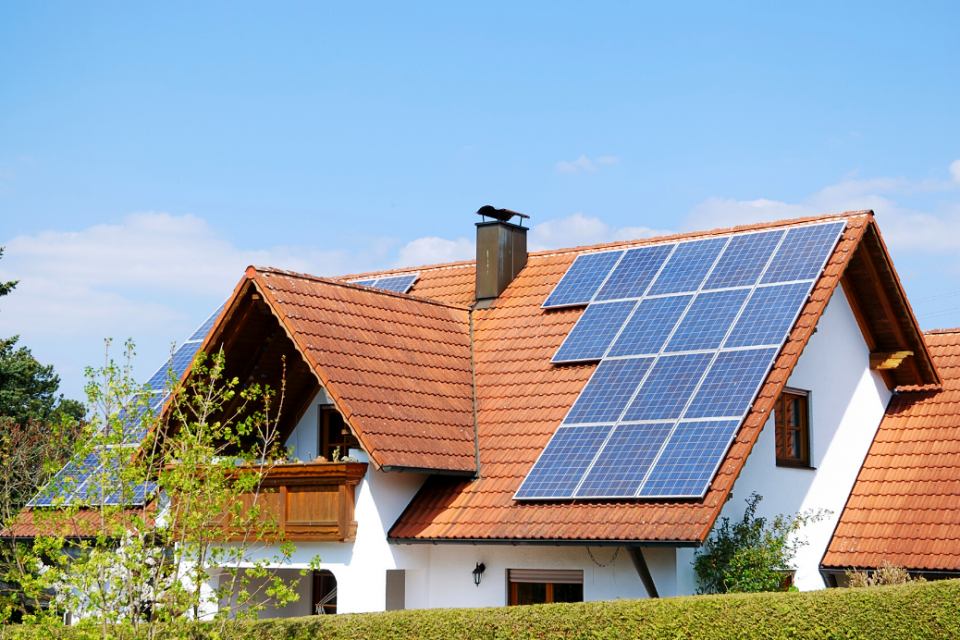# Shocking Truth: kW and kWh are Not the Same ThingEnergy consumption is a crucial aspect of our daily lives, and understanding the units of energy measurement is essential to manage it effectively. Kilowatts (kW) and kilowatt-hours (kWh) are two of the most commonly used units of energy, but they have distinct differences that are critical to understanding energy consumption.

#### Kilowatt (kW) vs. Kilowatt-hour (kWh)

A kilowatt (kW) is a unit of power, while a kilowatt-hour (kWh) is a unit of energy. Power is the rate at which energy is generated or consumed, while energy is the amount of power that is generated or consumed over a specific period.

To understand this difference better, consider an example of a car driving. The speedometer shows the speed at which the car is travelling at a particular moment, which is similar to the kW rating. The odometer shows the total distance travelled by the car over a specific period, which is similar to the kWh rating.

#### Solar PV Systems

Solar PV systems generate electricity from sunlight. The amount of electricity generated by a solar PV system depends on its capacity, which is measured in kilowatts (kW). For example, a 5 kW solar PV system can generate up to 5 kW of electricity at any given moment, provided there is sufficient sunlight.

The energy generated by a solar PV system over a period, such as a day or a month, is measured in kilowatt-hours (kWh). The kWh rating is essential to determine the size of the solar PV system needed to meet the energy needs of a household or business.

For instance, suppose a household requires 10 kWh of electricity per day. In that case, the size of the solar PV system needed to generate this amount of electricity depends on the location, the time of the year and the efficiency of the solar panels.

A larger solar PV system with a higher kW rating can potentially generate more electricity and therefore more kWh over a specific period of time. Understanding the difference between kW and kWh is essential to determine the size of the solar PV system needed to meet the energy needs of a household. More panels typically means a higher kW rating, which in turn translates to more kWh of electricity per year.

#### Heat Pumps

The energy consumption of a heat pump is measured in kilowatt-hours (kWh), which represents the amount of energy the heat pump consumes over a specific period. A higher kWh reading indicates that the heat pump has consumed more energy, while a lower kWh reading indicates that it has consumed less energy. Lower consumption equates to cheaper running costs.

Understanding the difference between kW and kWh is crucial to manage energy consumption effectively for heat pumps. The power rating of the heat pump (kW) determines the appropriate size of the heat pump required for the space being heated, which is determined by heat loss calculations.

kWh determine the overall energy consumption of the heat pump over a period, which can help to identify areas where energy efficiency can be improved. By measuring the energy consumption of the heat pump, it is possible to determine how much electricity is being used and identify ways to reduce energy usage and costs by playing around with weather compensation heat curves or flow temperatures.

If a heat pump is consuming a lot of energy (measured in kWh) over a specific period, it could be an indication that the heat pump is not operating efficiently or may require tweaks. By monitoring kWh readings, it is possible to optimise the operation of the heat pump and reduce energy consumption and costs.

#### Top Considerations When Choosing a Heat Pump for Your Home: Beginner’s Guide

Subscribe
Notify of